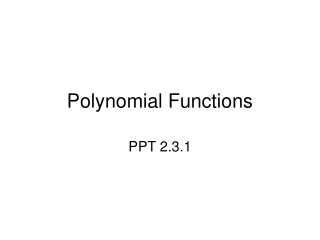DownloadDownload PresentationPolynomial Functions

# Polynomial Functions

Télécharger la présentation## Polynomial Functions

- - - - - - - - - - - - - - - - - - - - - - - - - - - E N D - - - - - - - - - - - - - - - - - - - - - - - - - - -
##### Presentation Transcript

1. Polynomial Functions PPT 2.3.1

2. Polynomial Functions The largest exponent within the polynomial determines the degree of the polynomial.

3. Explore Polynomials Linear Function Quadratic Function Cubic Function Quartic Function

4. Leading Coefficient The leading coefficient is the coefficient of the first term in a polynomial when the terms are written in descending order by degrees. For example, the quartic function f(x) = -2x4 + x3 – 5x2 – 10 has a leading coefficient of -2.

5. Graph A Graph B Cubic Polynomials Look at the two graphs and discuss the questions given below. 1. How can you check to see if both graphs are functions? • 2. How many x-intercepts do graphs A & B have? 3. What is the end behaviour for each graph? 4. Which graph do you think has a positive leading coeffient? Why? 5. Which graph do you think has a negative leading coefficient? Why?

6. Cubic Polynomials The following chart shows the properties of the graphs on the left.

7. Cubic Polynomials The following chart shows the properties of the graphs on the left.

8. Cubic Polynomials The following chart shows the properties of the graphs on the left.

9. Graph A Graph B Quartic Polynomials Look at the two graphs and discuss the questions given below. 1. How can you check to see if both graphs are functions? • 2. How many x-intercepts do graphs A & B have? 3. What is the end behaviour for each graph? 4. Which graph do you think has a positive leading coeffient? Why? 5. Which graph do you think has a negative leading coefficient? Why?

10. Quartic Polynomials The following chart shows the properties of the graphs on the left.

11. Quartic Polynomials The following chart shows the properties of the graphs on the left.

12. Quartic Polynomials The following chart shows the properties of the graphs on the left.

13. Quartic Polynomials The following chart shows the properties of the graphs on the left.